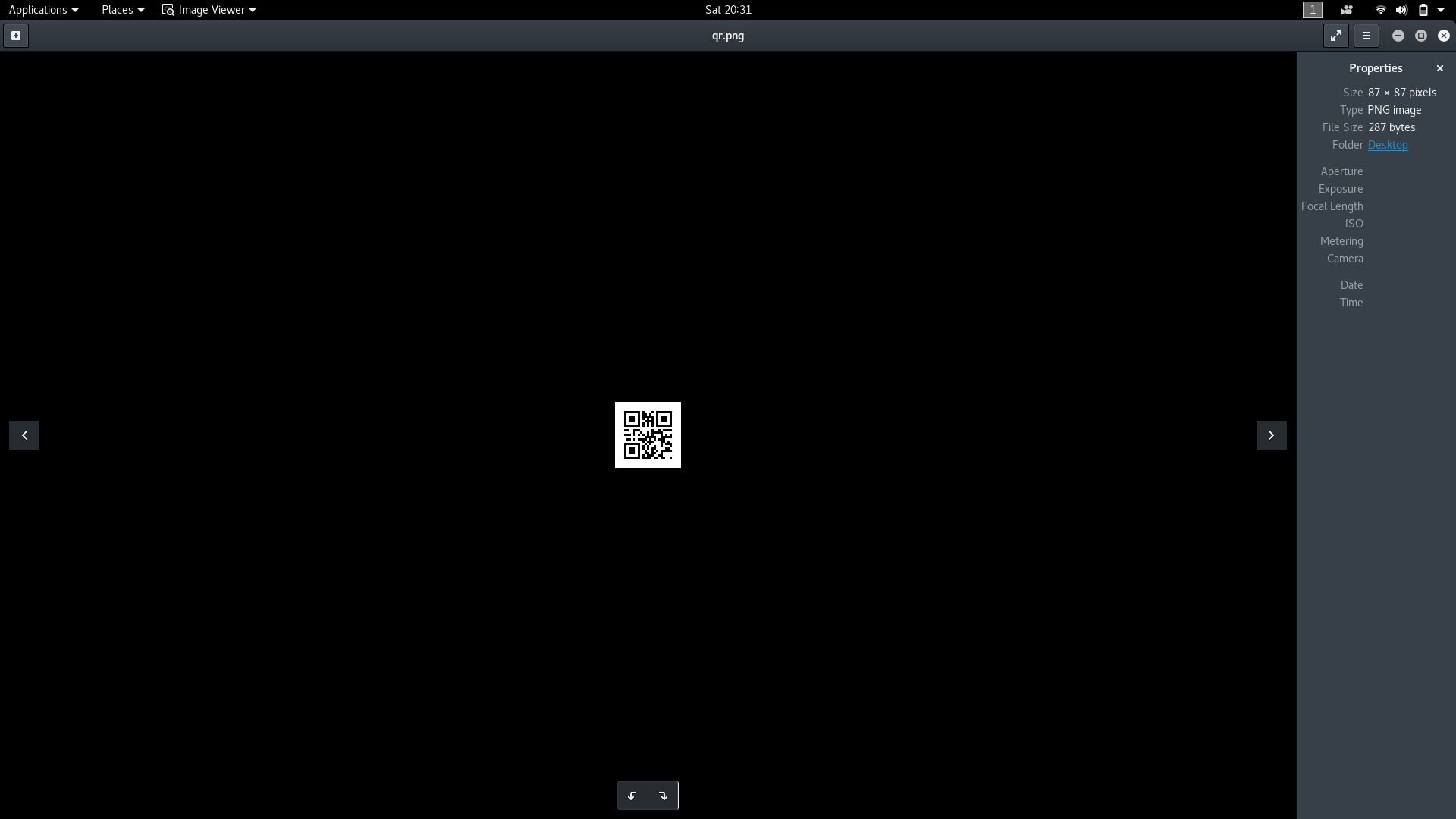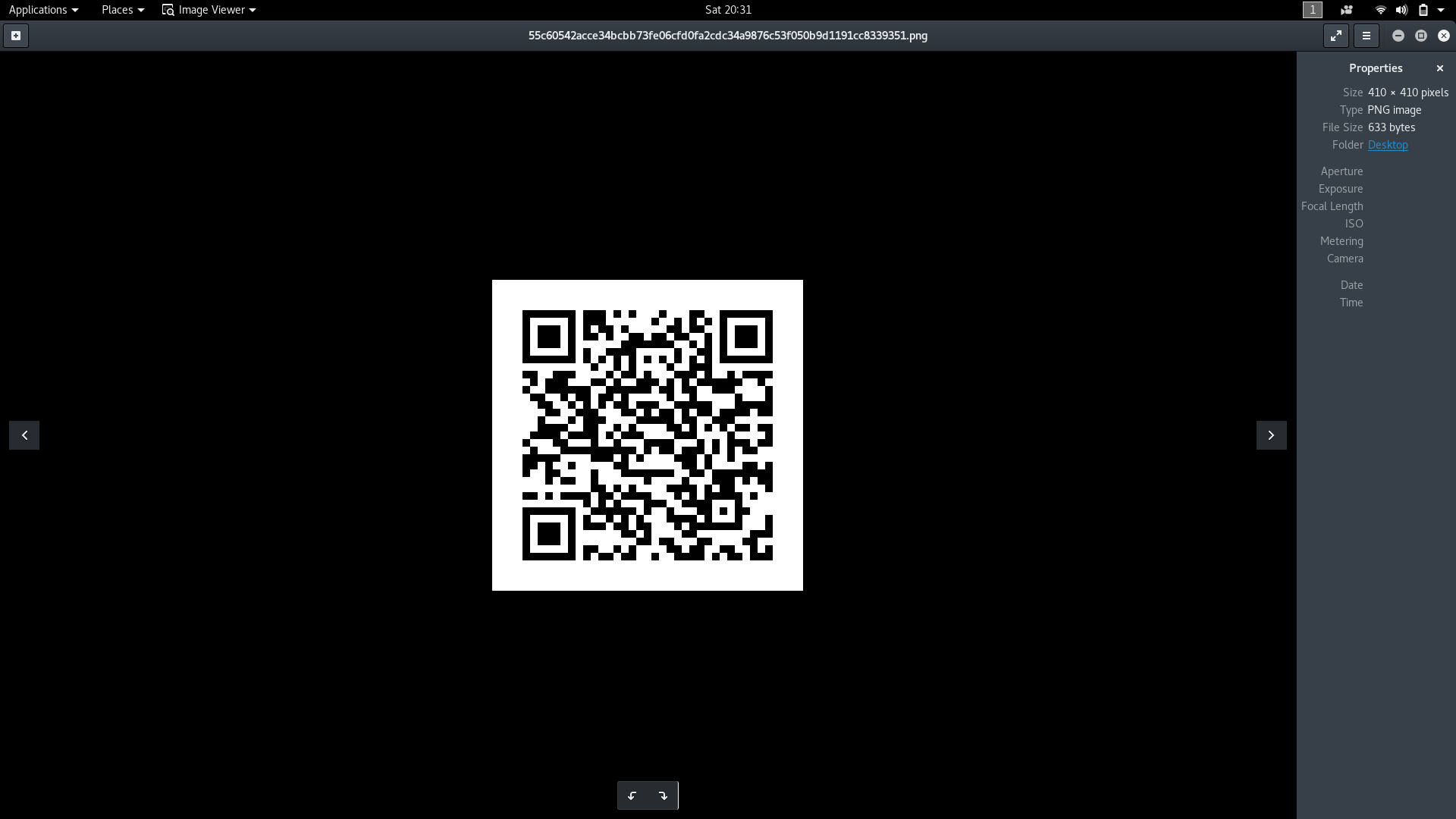Related Articles

# Reading and Generating QR codes in Python using QRtools

• Difficulty Level : Medium
• Last Updated : 22 Nov, 2020

This article aims to introduce the use of the python library: qrtools. This library can be used to both read QR codes and generate them.

What are QR codes?

QR code, or quick response code, is a trademark for a type of 2 dimensional barcode. 2 dimensional barcodes are similar to one dimensional barcodes, but can store more information per unit area.

Installation and Dependencies

1. Debian Linux: qrtools can be installed on debian based linux systems with the following commands
```sudo apt-get update
sudo apt-get install python-qrtools
```

The following dependencies must be installed as well

```[sudo] pip install pypng
[sudo] pip install zbar
[sudo] pip install pillow
```
```python setup.py install
```

Generate a QR Code

qrtools contains a class QR (can be viewed in the source code), for which we must initially create an object. The object takes the following arguments

1. data
2. pixel_size
3. level
4. margin_size
5. data_type

To create a QR code with default settings, we must simply specify the data while creating the object. Note that the data must be a unicode object if non-ASCII objects are going to be used.

 `# Python program to generate QR code``from` `qrtools``import` `QR`` ` `# creates the QR object``my_QR ``=` `QR(data ``=` `u``"Example"``)`` ` `# encodes to a QR code``my_QR.encode()`

If the program runs successfully, it returns a value of 0, and the QR code is stored in the tmp folder. To know the exact location, use the following command

```print (my_QR.filename)
```

Sample output

```/tmp/qr-1496334996.385343/7489ebbcc2a00056ddaaaac190bce473e5c03696ea1bd8ed83cf59a174283862.png
```This file can now be moved to another folder as per our convenience

 `# Python program to generate QR code``from` `qrtools ``import` `QR`` ` `import` `os``my_QR ``=` `QR(data ``=` `u``"Example"``)``my_QR.encode()`` ` `# command to move the QR code to the desktop``os.system(``"sudo mv "` `+` `my_QR.filename ``+` `" ~/Desktop"``)`

The pixel value of the QR code may also be changed by specifying the value during the creation of the QR object. The default size tends to be a little small for reading using scanners on smartphones, so a size of around 10 would be ideal for such purposes, for example:

```my_QR = QR(data = u"example", pixel_size = 10)
```

The below QR code has pixel size = 10, and has been encoded with a URLWe can also add email data, sms data, mms data, bookmarks, etc to the QR code. The following code excerpt is taken from the source code, which specifies the various datatypes that can be used along with the format of the data that would be required for its usage:

 `# use these for custom data formats eg. url, phone number, VCARD``# data should be an unicode object or a list of unicode objects``data_encode ``=` `{``    ``'text'``: ``lambda` `data: data,``    ``'url'``: encode_url,``    ``'email'``: ``lambda` `data: ``'mailto:'` `+` `re.``compile``(``        ``r``'^mailto:'``, re.IGNORECASE``    ``).sub('', data),``    ``'emailmessage'``: ``lambda` `data: ``'MATMSG:TO:'` `+` `data[``0``] ``+` `';SUB:'` `+` `data[``1``] ``+` `                           ``';BODY:'` `+` `data[``2``] ``+` `';;'``,``    ``'telephone'``: ``lambda` `data: ``'tel:'` `+` `re.``compile``(``        ``r``'^tel:'``, re.IGNORECASE``    ``).sub('', data),``    ``'sms'``: ``lambda` `data: ``'SMSTO:'` `+` `data[``0``] ``+` `':'` `+` `data[``1``],``    ``'mms'``: ``lambda` `data: ``'MMSTO:'` `+` `data[``0``] ``+` `':'` `+` `data[``1``],``    ``'geo'``: ``lambda` `data: ``'geo:'` `+` `data[``0``] ``+` `', '` `+` `data[``1``],``    ``'bookmark'``: ``lambda` `data: ``"MEBKM:TITLE:"` `+` `data[``0``] ``+` `";URL:"` `+` `                                    ``data[``1``] ``+` `";;"``,``    ``# phonebook or meCard should be a list of tuples like this:``    ``# [('N', 'Name'), ('TEL', '231698890'), ...]``    ``'phonebook'``: ``lambda` `data: ``"MECARD:"` `+` `";"``.join([``":"``.join(i) ``                                        ``for` `i ``in` `data]) ``+` `";"``}`

From the above code, we observe the various data types that can be assigned and used while creating QR codes. For example, to use a bookmark as data we must provide data as a list, consisting of a title and the url. To accomplish this, we must do the following

 `# Python program to generate QR code``from` `qrtools ``import` `QR`` ` `# creates the QR object``my_QR ``=` `QR(data ``=` `[u``"geeksforgeeks"``, u``"https://www.geeksforgeeks.org/"``], ``                                      ``data_type ``=` `'bookmark'``)`` ` `# encodes to a QR code``my_QR.encode()`

Scanning and reading a QR code is relatively simple. While creating the QR object, we must simply specify the path to the QR code as an argument. Suppose we are trying to decode the QR code created at the beginning of the article.

 `# Python program to Scan and Read a QR code``from` `qrtools ``import` `QR``my_QR ``=` `QR(filename ``=` `"home/user/Desktop/qr.png"``)`` ` `# decodes the QR code and returns True if successful``my_QR.decode()`` ` `# prints the data``print` `(my_QR.data)`

Output :

```Example
```We may also print the values of the other parameters passed while creating the QR object to generate the QR code, for example, using the same QR code generated at the beginning of the article, additionally adding these print statements would give the following additional output

```print (my_QR.data_type)
print (my_QR.pixel_size)
print (my_QR.margin_size)
```

Output:

```text
3
4
```This article is contributed by Deepak Srivatsav. If you like GeeksforGeeks and would like to contribute, you can also write an article using contribute.geeksforgeeks.org or mail your article to contribute@geeksforgeeks.org. See your article appearing on the GeeksforGeeks main page and help other Geeks.